Normal view MARC view ISBD view

# A course in functional analysis and measure theory / Vladimir Kadets ; translated from the Russian by Andrei Iacob.

Material type:TextSeries: Universitext: Publisher: Cham, Switzerland : Springer, 2018Description: xxii, 539 pages, E 54.99 23 cms.Content type: text Media type: computer Carrier type: online resourceISBN: 9783319920047; 3319920049.Genre/Form: Electronic books.DDC classification: 515/.7
Contents:
Introduction -- Chapter 1. Metric and topological spaces -- Chapter 2. Measure theory -- Chapter 3. Measurable functions -- Chapter 4. The Lebesgue integral -- Chapter 5. Linear spaces, linear functionals, and the Hahn-Banach theorem -- Chapter 6. Normed spaces -- Chapter 7. Absolute continuity of measures and functions. Connection between derivative and integral -- Chapter 8. The integral on C(K) -- Chapter 9. Continuous linear functionals -- Chapter 10. Classical theorems on continuous operators -- Chapter 11. Elements of spectral theory of operators. Compact operators -- Chapter 12. Hilbert spaces -- Chapter 13. Functions of an operator -- Chapter 14. Operators in Lp -- Chapter 15. Fixed-point theorems and applications -- Chapter 16. Topological vector spaces -- Chapter 17. Elements of duality theory -- Chapter 18. The Krein-Milman theorem and applications -- References. Index.
List(s) this item appears in: 2019-04-30
Item type Current location Call number Status Date due Barcode Item holds
Book Chennai Mathematical Institute
General Stacks
515.7 KAD (Browse shelf) Available 10641
Total holds: 0
##### Browsing Chennai Mathematical Institute Shelves , Shelving location: General Stacks Close shelf browser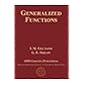No cover image available No cover image available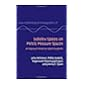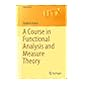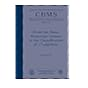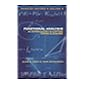515.7 GEL Generalized functions / Vol. 6 representation theory and automorphic functions. 515.7 HAA Functional analysis : an elementary introduction / 515.7 HEI Sobolev spaces on metric measure spaces : an approach based on upper gradients / 515.7 KAD A course in functional analysis and measure theory / 515.7 LIN From the basic homotopy lemma to the classification of C*-algebras / 515.7 Mac Elementary functional analysis / 515.7 STE Functional analysis : introduction to further topics in analysis /

Includes bibliographical references and index.

Introduction -- Chapter 1. Metric and topological spaces -- Chapter 2. Measure theory -- Chapter 3. Measurable functions -- Chapter 4. The Lebesgue integral -- Chapter 5. Linear spaces, linear functionals, and the Hahn-Banach theorem -- Chapter 6. Normed spaces -- Chapter 7. Absolute continuity of measures and functions. Connection between derivative and integral -- Chapter 8. The integral on C(K) -- Chapter 9. Continuous linear functionals -- Chapter 10. Classical theorems on continuous operators -- Chapter 11. Elements of spectral theory of operators. Compact operators -- Chapter 12. Hilbert spaces -- Chapter 13. Functions of an operator -- Chapter 14. Operators in Lp -- Chapter 15. Fixed-point theorems and applications -- Chapter 16. Topological vector spaces -- Chapter 17. Elements of duality theory -- Chapter 18. The Krein-Milman theorem and applications -- References. Index.

Online resource; title from PDF title page (SpringerLink, viewed July 23, 2018).